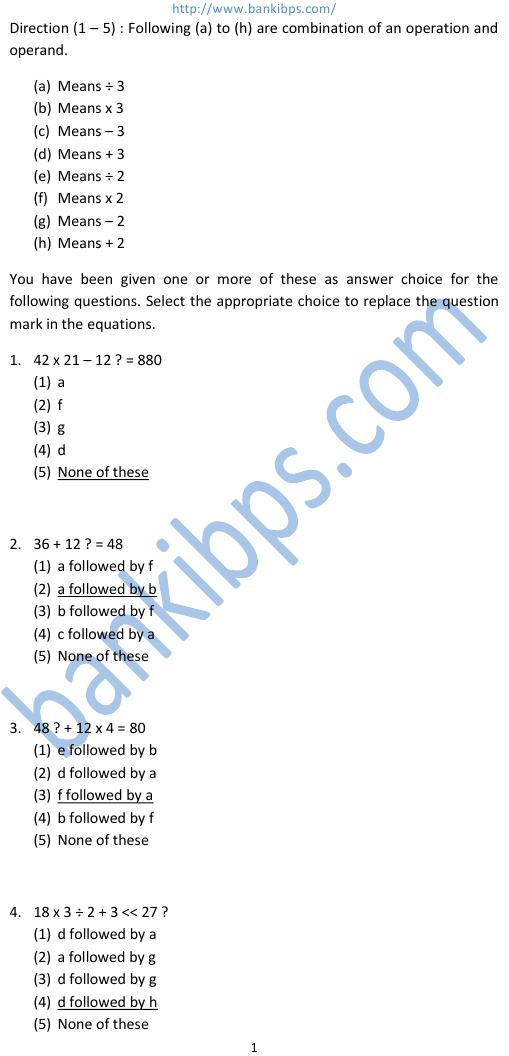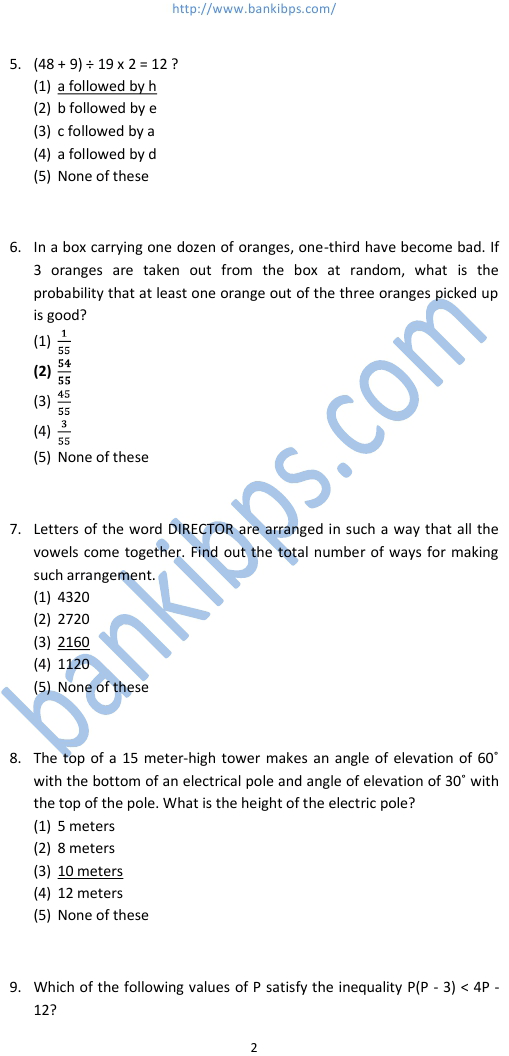# Bank Aptitude Test Questions

Direction (1 – 5) : Following (a) to (h) are combination of an operation and operand. bank aptitude test questions (a) Means ÷ 3 (b) Means x 3 (c) Means – 3 (d) Means + 3 (e) Means ÷ 2 (f) Means x 2 (g) Means – 2 (h) Means + 2 You have been given one or more of these as answer choice for the following questions. Select the appropriate choice to replace the question mark in the equations. 1. 42 x 21 – 12 ? = 880 (1) a (2) f (3) g (4) d (5) None of these 2. 36 + 12 ? = 48 (1) a followed by f (2) a followed by b (3) b followed by f (4) c followed by a (5) None of these 3. 48 ? + 12 x 4 = 80 (1) e followed by b (2) d followed by a (3) f followed by a (4) b followed by f (5) None of these 4. 18 x 3 ÷ 2 + 3 (1) d followed by a (2) a followed by g (3) d followed by g (4) d followed by h (5) None of these
Practice Exercise - 327 [ Aptitude ]## bank aptitude test questions

### bank aptitude test questions and answers pdf

#### aptitude test questions answers banks

##### aptitude questions for bank po exam
###### aptitude test questions and answers for banks
bank aptitude test questions and answers pdf. aptitude test questions answers banks. aptitude questions for bank po exam. aptitude test questions and answers for banks. aptitude test for bank exam. bank aptitude test questions and answer. bank aptitude test questions. bank exam aptitude question. bank exam aptitude question and answer. aptitude test tricks. aptitude questions and answers with explanation. clerical aptitude questions and answers for bank exam.